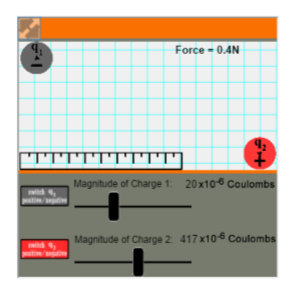# 5.10: Coulomb’s Law

$$\newcommand{\vecs}{\overset { \rightharpoonup} {\mathbf{#1}} }$$ $$\newcommand{\vecd}{\overset{-\!-\!\rightharpoonup}{\vphantom{a}\smash {#1}}}$$$$\newcommand{\id}{\mathrm{id}}$$ $$\newcommand{\Span}{\mathrm{span}}$$ $$\newcommand{\kernel}{\mathrm{null}\,}$$ $$\newcommand{\range}{\mathrm{range}\,}$$ $$\newcommand{\RealPart}{\mathrm{Re}}$$ $$\newcommand{\ImaginaryPart}{\mathrm{Im}}$$ $$\newcommand{\Argument}{\mathrm{Arg}}$$ $$\newcommand{\norm}{\| #1 \|}$$ $$\newcommand{\inner}{\langle #1, #2 \rangle}$$ $$\newcommand{\Span}{\mathrm{span}}$$ $$\newcommand{\id}{\mathrm{id}}$$ $$\newcommand{\Span}{\mathrm{span}}$$ $$\newcommand{\kernel}{\mathrm{null}\,}$$ $$\newcommand{\range}{\mathrm{range}\,}$$ $$\newcommand{\RealPart}{\mathrm{Re}}$$ $$\newcommand{\ImaginaryPart}{\mathrm{Im}}$$ $$\newcommand{\Argument}{\mathrm{Arg}}$$ $$\newcommand{\norm}{\| #1 \|}$$ $$\newcommand{\inner}{\langle #1, #2 \rangle}$$ $$\newcommand{\Span}{\mathrm{span}}$$graph sourceimage source

As seen in the section on orbitals, the energy of an electron in an isolated hydrogen atom is determined by the principal quantum number n. The reason for this is not exactly the same as the reason that the energy of the particle in a box depended on a whole number n, however. In an atom an electron has both kinetic energy (like the particle in a box) and potential energy. The electron’s potential energy is a result of the attractive force between the negatively charged electron and the positively charged nucleus.

When unlike charges (one negative and the other positive) attract each other, or like charges (both positive or both negative) repel each other, Coulomb’s law governs the force between them. According to this law the force of attraction or repulsion varies inversely with the square of the distance between the charges. Suppose two particles, one with a charge of +1 μC (microcoulomb) and the other with a charge of –1 μC are placed 1cm apart. The force of attraction between these two charges is found to be 90 N (newton), about the same force as gravity exerts on a 20-lb weight. If the distance between the charges is now multiplied by a factor of 100 (increased to 1 m), then the force of attraction between the two charges is found to be divided by a factor of 100 squared, i.e., by a factor of 104. Consequently the force decreases to about the weight of a grain of sand. Another hundredfold increase in the distance (to 100 m or about 100 yd) reduces the force of attraction by a further factor of 104, making the force virtually undetectable.

As the above example shows, electrostatic forces of attraction and repulsion are very strong when the charges are close, but drop off fairly quickly as the charges are separated.

If the separation is very large, electrostatic forces can often be neglected. This behavior resembles the more familiar example of attraction and repulsion between the poles of magnets and also is akin to the force of gravity in the solar system. Magnetic forces and gravitational forces follow an inverse square relationship as well.

A second part of Coulomb’s law states that the force is proportional to the magnitude of each charge. In the above example if one charge is doubled (to ±2 μC), the force is likewise doubled, while if both charges are doubled, the force is multiplied by four. Coulomb’s law is summarized by the equation

$F=k\frac{Q_{1}Q_{2}}{r^{2}}$

where F is the force, Q1 and Q2 are the charges, and r is the distance between the charges. The constant k has the value 8.988 × 109 N m2 C–2.

To see this equation in action, check out the following simulation: